#DenseNet的应用--语义分割--(DenseASPP )

0
0
01. 云栖社区>
2. 博客>
3. 正文

## DenseNet的应用--语义分割--(DenseASPP )

ayew 2018-07-15 10:18:00 浏览1942

# DenseASPP

DenseASPP for Semantic Segmentation in Street Scenes

# Introduction

## ASPP模块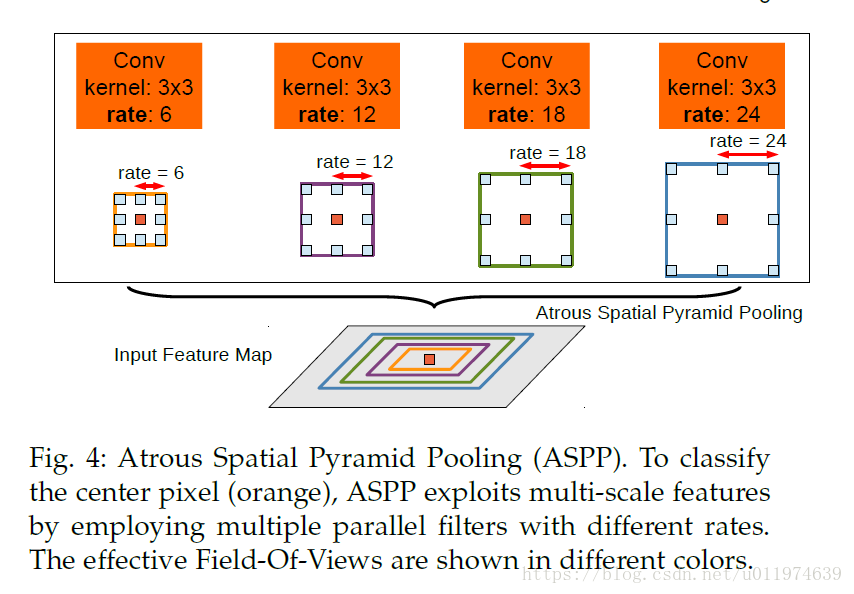## DenseNet

DenseNet中用密集连接获得更加的性能：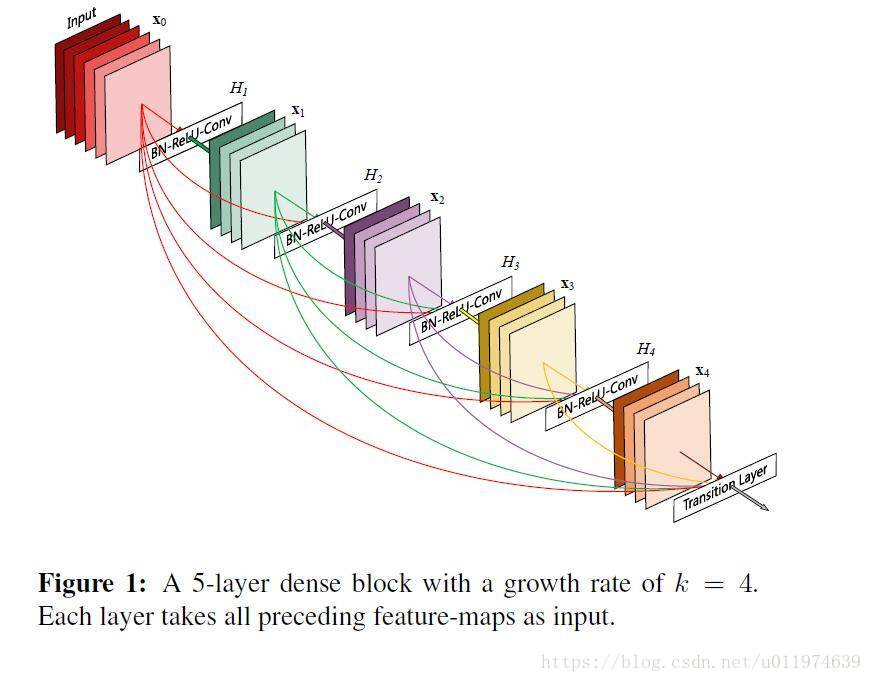## DenseASPP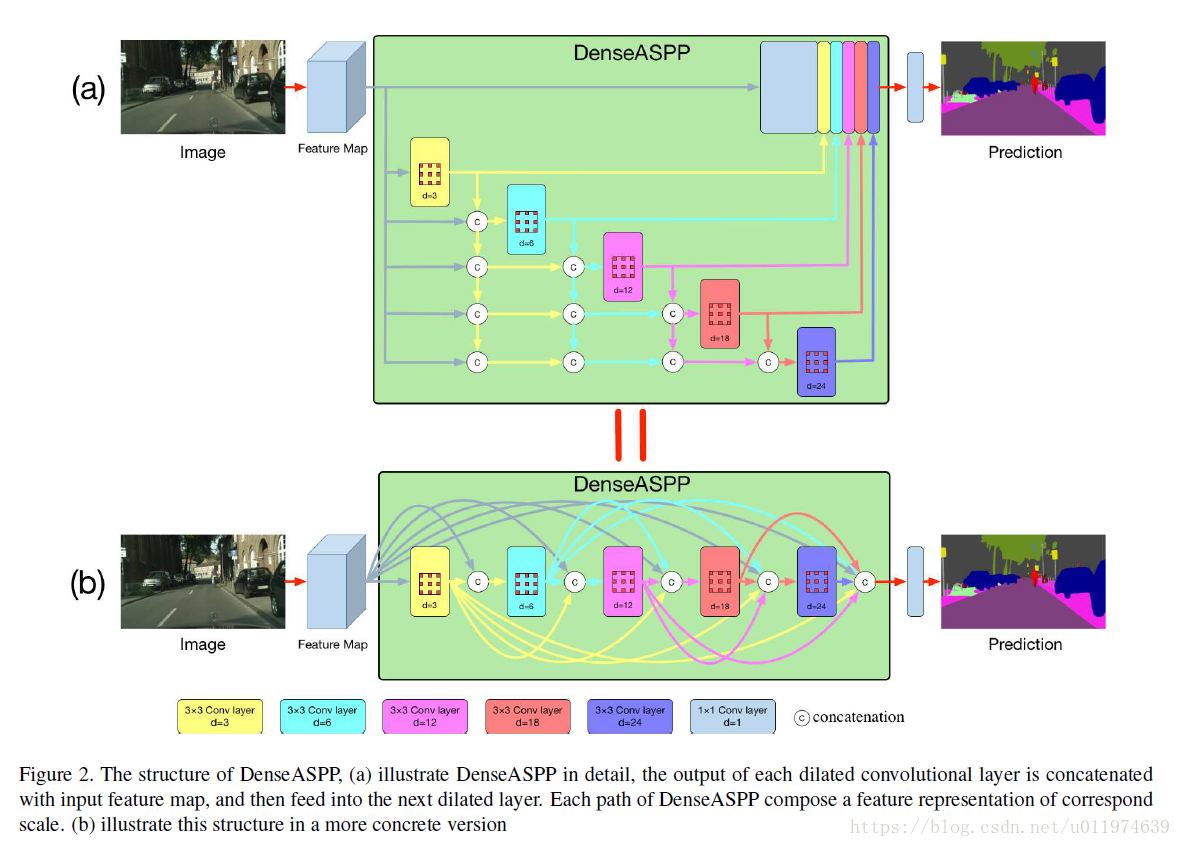• DenseASPP能够生成覆盖非常大的范围的接收野特征
• DenseASPP能够以非常密集的方式生成的特征

## Related Work

DenseASPP是DenseNet的特例，可以看做是所有扩张率设置为1，这分享了DenseNet的优点，包括缓解了梯度消失的问题和大幅度减少参数。

# Dense Atrous Spatial Pyramid Pooling

• backbone:级联的扩张卷积（这和DRN，HDC是一个思想），逐渐获取到大的感受野特征
• ASPP：同一输入上并行的扩张卷积组合，将输出级联到一起

y=H3,6(x)+H3,12(x)+H3,18(x)+H3,24(x)y=H3,6(x)+H3,12(x)+H3,18(x)+H3,24(x)

## Denser feature pyramid and larger receptive field

DenseASPP的结构如下：yl=HK,,dl([yl1,yl2,...,y0])yl=HK,,dl([yl−1,yl−2,...,y0])

• 密集的特征金字塔(denser feature pyramid)
• 更大的接收野(larger receptive field.)

### Denser feature pyramid

R=(d1)×(K1)+KR=(d−1)×(K−1)+K

K=K1+K21K=K1+K2−1

DenseASPP由包含扩张率为3,6,12,183,6,12,18的扩张卷积，每组数字的表示扩张率的组合，长度表示等效的卷积核大小，kk表示实际的接收野，如下所示：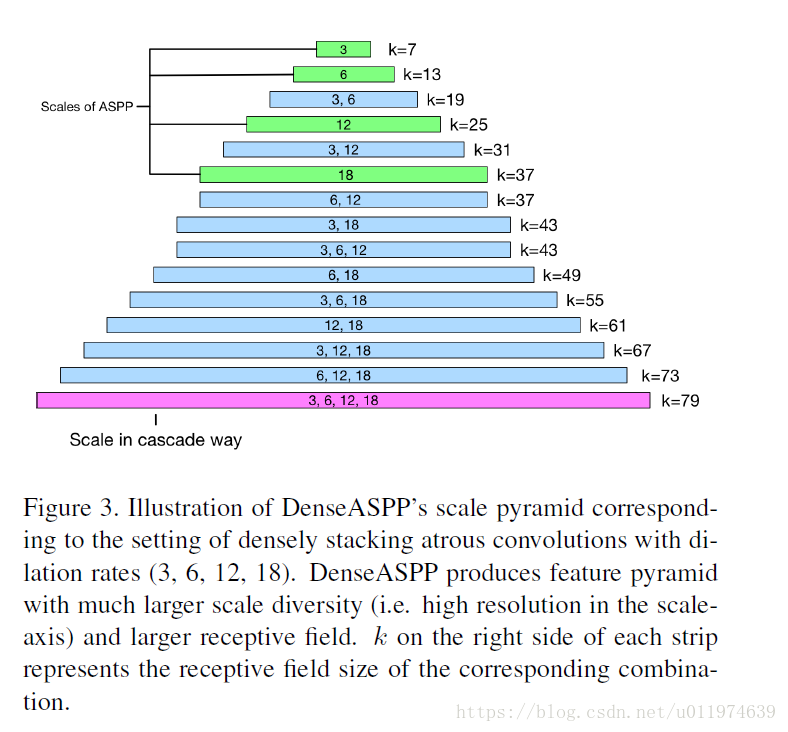• K=3,d=3(31)×(31)+3=7(3−1)×(3−1)+3=7
• K=3,d=6(61)×(31)+3=13(6−1)×(3−1)+3=13
• K=3,d=3,d=6
• 第一个扩张卷积：(31)×(31)+3=7(3−1)×(3−1)+3=7
• 第二个扩张卷积：(61)×(31)+3=13(6−1)×(3−1)+3=13
• 组合：7+131=197+13−1=19
• K=3,d=12(121)×(31)+3=25(12−1)×(3−1)+3=25
• K=3,d=3,127+251=317+25−1=31
• K=3,d=3,6,127+13+252=437+13+25−2=43
• K=3,d=3,6,12,187+13+25+373=797+13+25+37−3=79

### 更密集的采样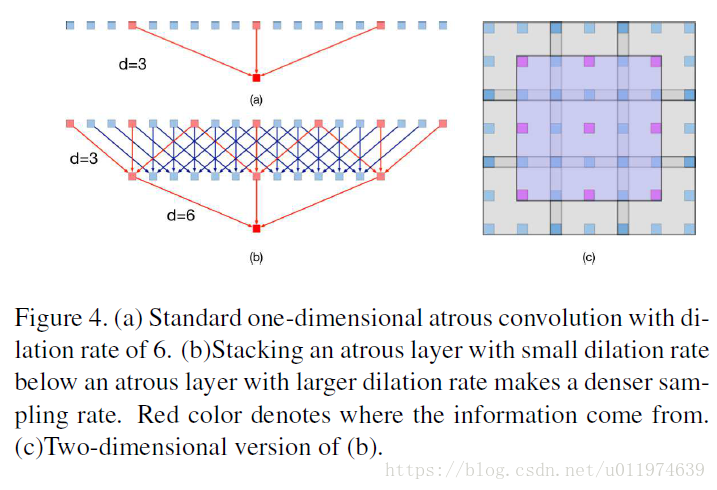### Larger receptive field

Rmax=max[R3,6,R3,12,R3,18,R3,24]$=R3,24=51Rmax=max[R3,6,R3,12,R3,18,R3,24]$=R3,24=51

Rmax=R3,6+R3,12+R3,18+R3,243=122Rmax=R3,6+R3,12+R3,18+R3,24−3=122

## Model size control

cl=c0+n×(l1)cl=c0+n×(l−1)

S=l=1L[cl×12×c02+c02×K2×n]=l=1L[c02(c0+(l1)×c08)+c02×K2×c08]=c208(15+L+2K2)LS=∑l=1L[cl×12×c02+c02×K2×n]=∑l=1L[c02(c0+(l−1)×c08)+c02×K2×c08]=c028(15+L+2K2)L

# Experiment

## DenseASPP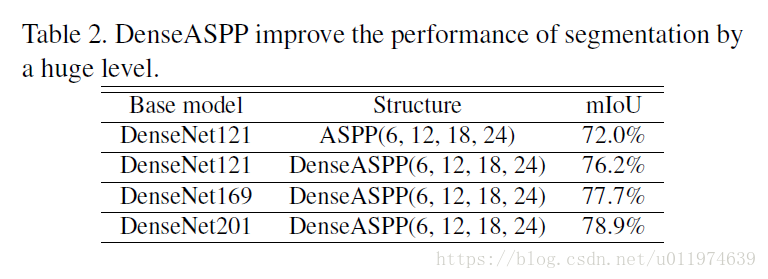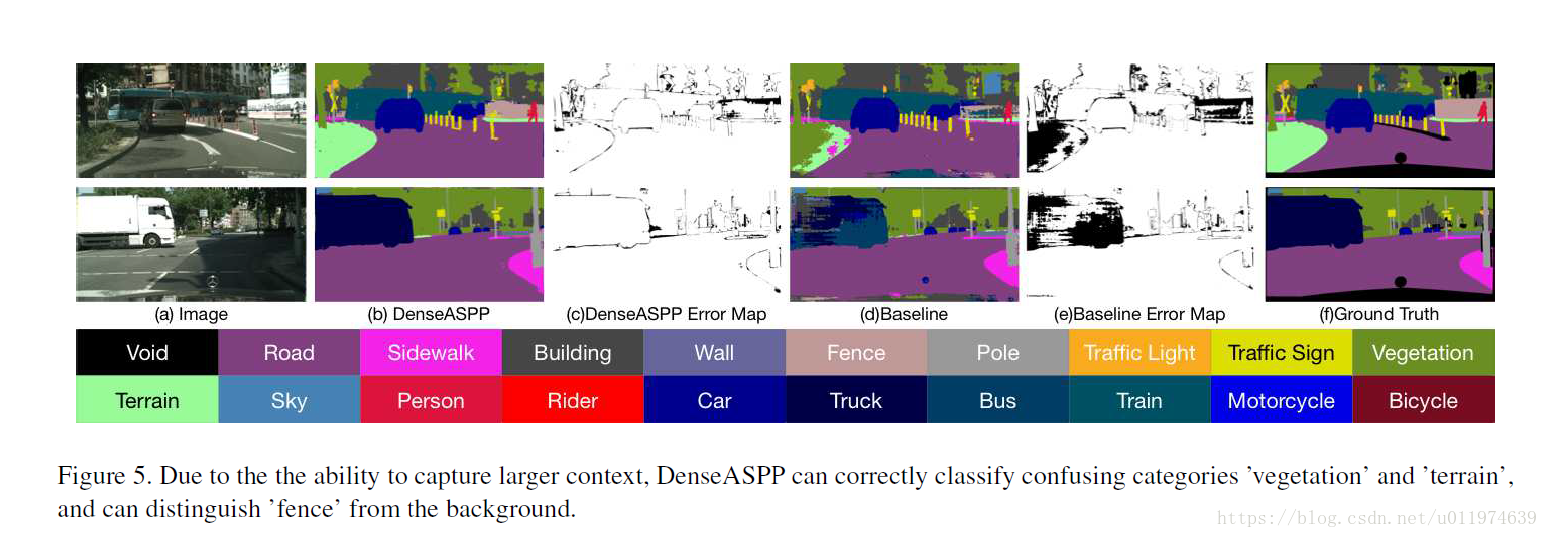## Detailed study on DenseASPP components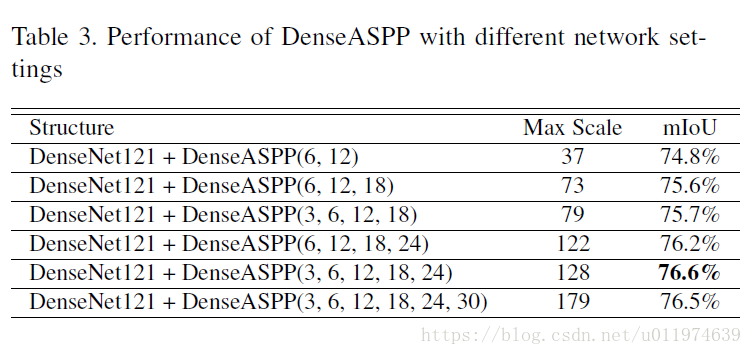## Comparing with state-of-the-art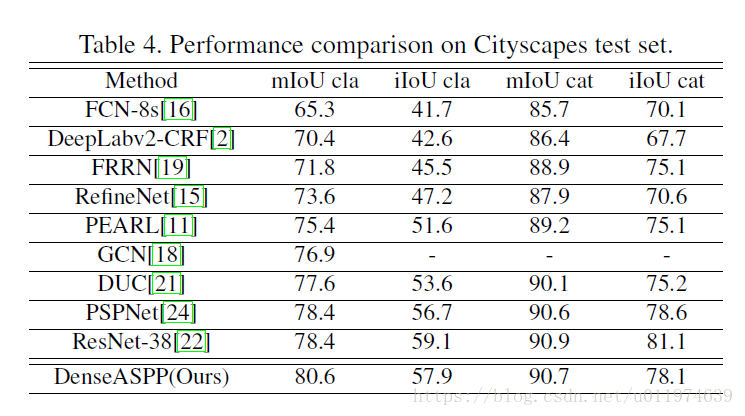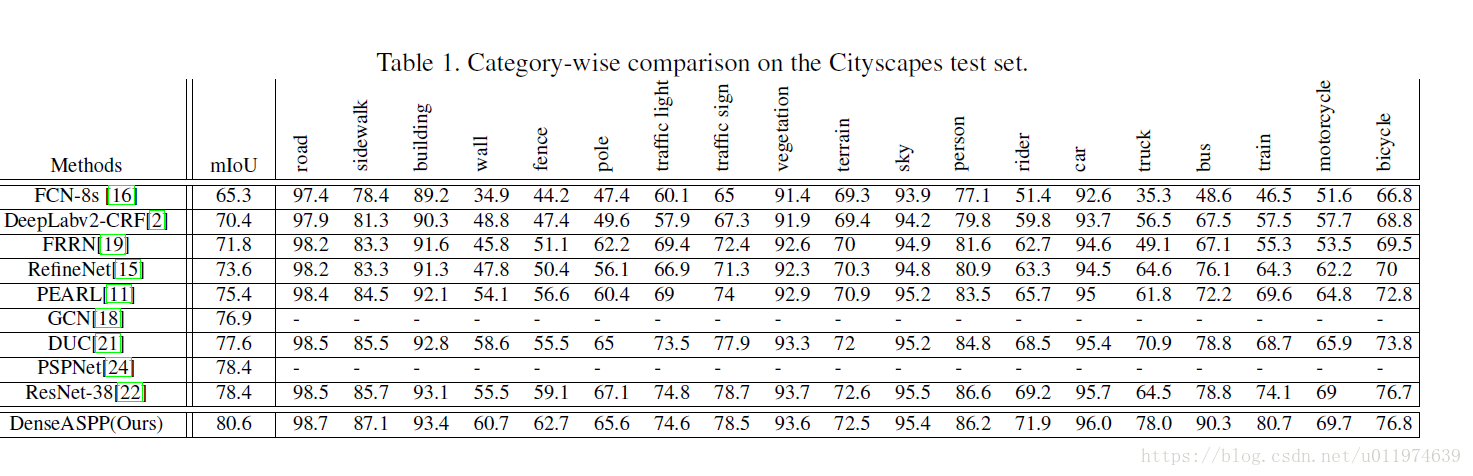## Ablation Studies

### Feature similarities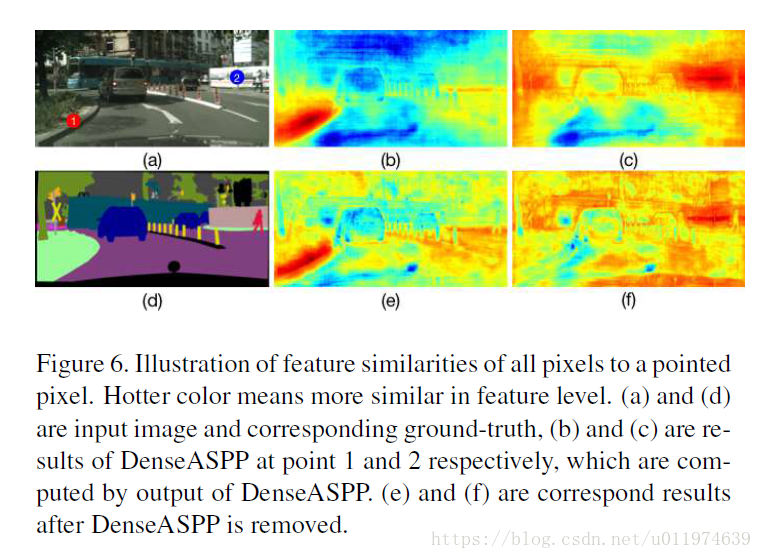### Visualization of receptive field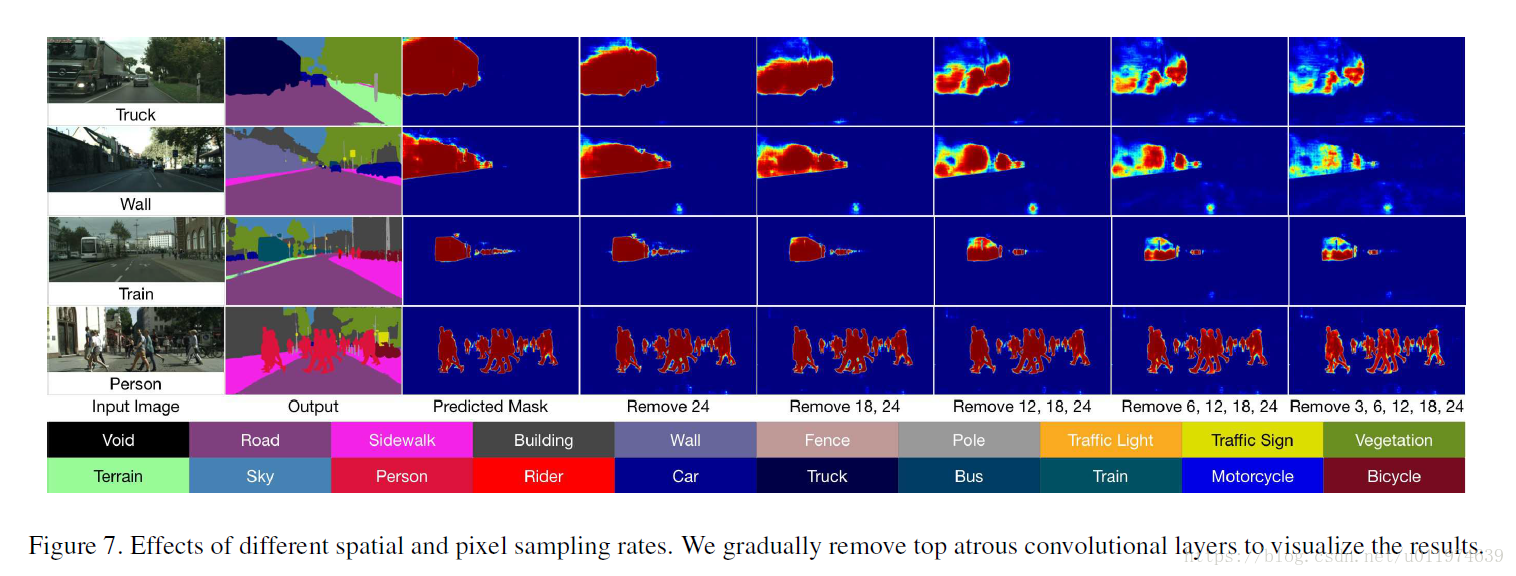DenseASPP和ASPP对比的接收野如下：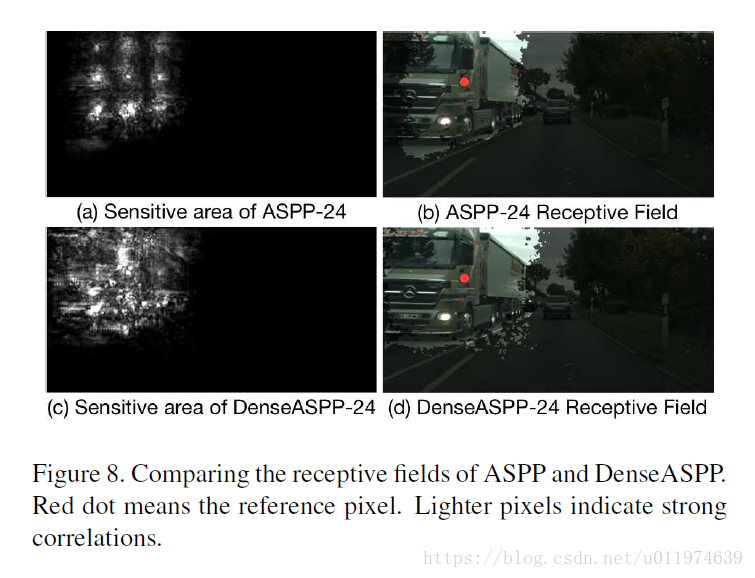# Conclusion

DenseASPP能够以更密集的方式连接一组扩张卷积，可以在很大范围内有效的生成密集的空间采样和特征，在CityScapes上测试得到了state-of-the-art的结果。

ayew
+ 关注